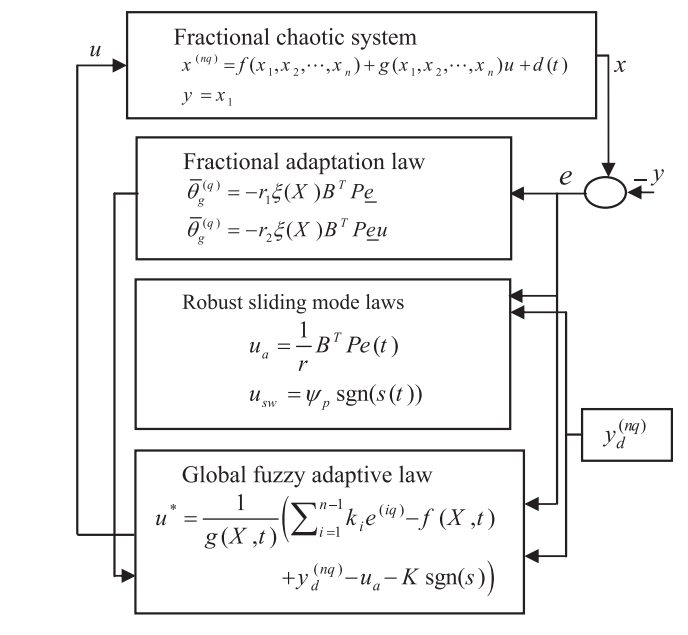Book Chapter

# Enhanced Fractional Order Indirect Fuzzy Adaptive Synchronization of Uncertain Fractional Chaotic Systems Based on the Variable Structure Control : Robust H ∞ Design Approach

By
Khettab K.
Bensafia Y.
Bourouba B.
Azar A.T.

This work presents a novel fractional H8 robust indirect adaptive fuzzy logic control strategy based on the variable structure control theory design (FRAFC-VSC) to synchronize two fractional-order chaotic systems. The contribution of this work is the use of an adaptive fractional order PI-regulator and a saturation function to eliminate the chattering phenomena in the control and surface signals. Stability analysis is performed for the proposed method with an acceptable synchronization error level based on Lyapunov stability criterion. The synchronization of two different fractional order chaotic systems is used to demonstrate the performance of the proposed fractional fuzzy adaptive scheme. Simulations are implemented using a numerical method based on Grünwald-Letnikov approximation approach (G-L) to solve the fractional differential equations. Numerical simulations are done to show the effectiveness of the proposed method. © 2018 Elsevier Inc. All rights reserved.